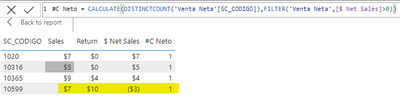cancel
Showing results for
Did you mean:Frequent Visitor

## Help using CALCULATE and FILTER

Hello

I am trying to use a distinct count using CALCULATE and FILTER but for some reason is not working:

Measure "\$ Net Sales" is = Sales - Return and is working fine, but when I try to count customers (SC_CODIGO) which "\$ Net Sales" is > 0 it counts customers which Sales is > 0, not net sales.1 ACCEPTED SOLUTIONSuper User

Hmm. That's strange unless [Return] and [Sales] are measures too. (I was assuming they were columns.)

If they are measures, then I'd recommend a different approach:

``````#C Net =
SUMX (
DISTINCT ( 'Venta Neta'[SC_CODIGO] ),
IF ( [Return] > [Sales], 1 )
)``````
4 REPLIES 4Super User

You have to be careful using measures inside of a FILTER since the measure is evaluated after a row context to filter context transition.

I'd recommend avoiding it where you can and instead write:

``````#C Net =
CALCULATE (
DISTINCTCOUNT ( 'Venta Neta'[SC_CODIGO] ),
FILTER ( 'Venta Neta', 'Venta Neta'[Return] > 'Venta Neta'[Sales] )
)``````Frequent Visitor

Thank you Alexis for your help, now "#C Neto" column is ok, but the total number is counting all rows, it shoud be 669 (which have 1 in #C Neto column) and not 749.RegardsSuper User

Hmm. That's strange unless [Return] and [Sales] are measures too. (I was assuming they were columns.)

If they are measures, then I'd recommend a different approach:

``````#C Net =
SUMX (
DISTINCT ( 'Venta Neta'[SC_CODIGO] ),
IF ( [Return] > [Sales], 1 )
)``````Frequent Visitor

Thank you very much for your help! now is working!

Regards